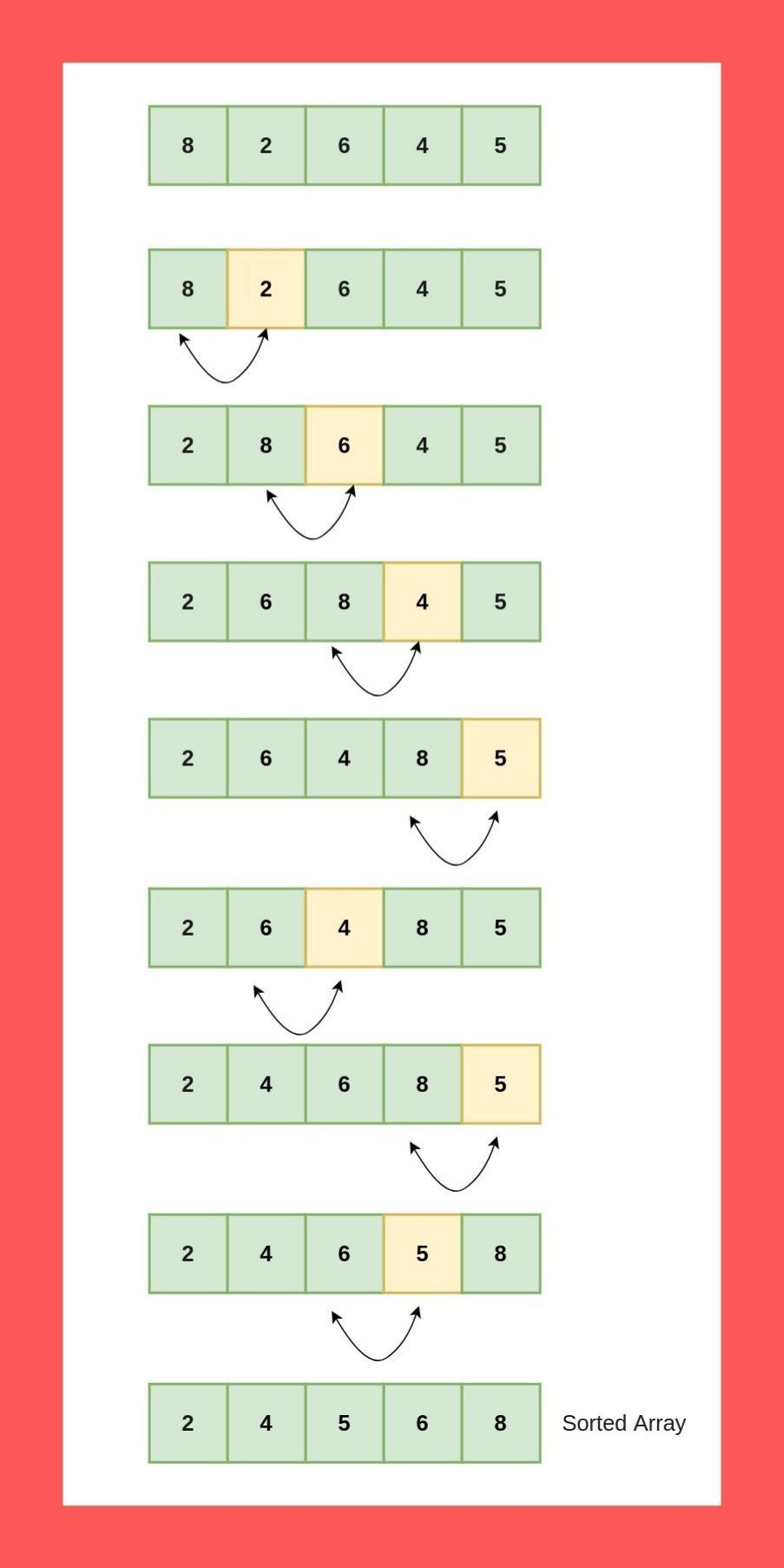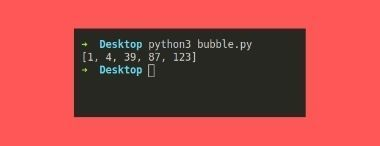Bubble sort is the simplest sorting algorithm in computer science. Bubble sort performs the sorting process by swapping adjacent elements if they’re not in the desired (ascending or descending) order. This process repeats from the beginning of the list of elements until all elements are in order.

In order to understand the sorting process, let’s go through this explanation picture.The array contains five-element and we are using the Bubble sort to perform the sorting in ascending order. As you can see, in each iteration, we are checking if the adjacent elements are in order. We are checking the left side element is greater than the right side element and if they are, we are swapping them. After doing all the swaps, in the end we get the sorted array.

Let’s implement it in Python.

## Bubble Sort in Python

Here is the sample code, please do copy it and paste it into the file naming bubble.py.

def bubble_sort(array):
n = len(array)

# first loop to iterate through the array from 0th element
for i in range(n):
# flag to terminate the process if there is nothing left to sort

# Second loop to check the adjacent element
for j in range(n - i - 1):
if array[j] > array[j + 1]:
# swap the elements
array[j], array[j + 1] = array[j + 1], array[j]